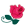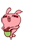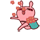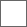### 【签子铺】默然转身，乱世的帷幕，砰然落下。。。

【这个是昨晚做的签 整体·总之还行吧】
【作品啥的】【顺便喂一下度娘~】
催单者不接裸奔不接太难的饶了我【大神们绕道吧~】长期招收电源，欢迎报名~~

=电源招聘=
=ID=
=昵称=（好记点的行不？我记性不好）
=会做神马=
=作品=
=弃店否==古风签格式=
=图片=（可多张）
=文字=
=风格=
=署名=0u00v0 什么就算太难楼上的如果需要的话 可以先交表
白子先试试看 做不出来的就算太难【白子是一只小菜鸟啦】=图片==文字=可人如玉、与子偕臧，长亭远望、人走茶凉。
=风格=悲伤唯美
=署名=小狐百度所接受的签名档最大格式为500*200

=古风签格式=
=图片=（可多张）=文字=断了弓弦，断了心跳，断了浮生，望断缥缈。
=风格=随意
=署名=炙
谢谢楼主~

格式：
=古风签格式=
=图片=
=文字=取次花丛懒回顾，半缘修道半缘居。
=风格=不知道。
=署名=崎

百度小说人气榜

#### 扫二维码下载贴吧客户端

下载贴吧APP
看高清直播、视频！